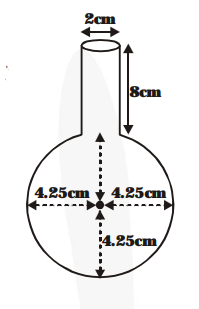# A spherical glass vessel has a cylindrical neck 8 cm long,

Question:

A spherical glass vessel has a cylindrical neck $8 \mathrm{~cm}$ long, $2 \mathrm{~cm}$ in diameter; the diamter of the spherical part is $8.5 \mathrm{~cm}$. By measuring the amount of water it holds, a child finds its volume to be $345 \mathrm{~cm}^{3}$. Check whether she is correct, taking the above as the inside measurements, and $\pi$ $=3.14$.

Solution:

The cylinder neck has length = 8 cm

Volume of the cylinder part

$=\pi(1)^{2} \times 8 \mathrm{~cm}^{3}$

$=8 \pi \mathrm{cm}^{3}$

The radius of the spherical part$=\frac{85}{2} \mathrm{~cm}=4.25 \mathrm{~cm}$

Volume of the spherical part

$=\frac{\mathbf{4}}{\mathbf{3}} \pi \times(4.25)^{3}$

Total volume of water

$=8 \pi+\frac{\mathbf{4}}{\mathbf{3}} \times(4.25)^{3} \pi \mathrm{cm}^{3}$

$=8 \times 3.14+\frac{\mathbf{4}}{\mathbf{3}} \times 3.14 \times(4.25)^{3} \mathrm{~cm}^{3}$

$=25.12+321.38$ (approx.)

$=346.5 \mathrm{~cm}^{3}$ (approx.)

So, $345 \mathrm{~cm}^{3}$ is not correct.